Journal topic
Magn. Reson., 1, 175–186, 2020
https://doi.org/10.5194/mr-1-175-2020Magn. Reson., 1, 175–186, 2020
https://doi.org/10.5194/mr-1-175-2020Research article 07 Aug 2020

Research article | 07 Aug 2020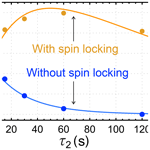# Geminal parahydrogen-induced polarization: accumulating long-lived singlet order on methylene proton pairs

Geminal parahydrogen-induced polarization: accumulating long-lived singlet order on methylene proton pairs
Laurynas Dagys1,, Barbara Ripka1,, Markus Leutzsch2, Gamal A. I. Moustafa1, James Eills3, Johannes F. P. Colell1, and Malcolm H. Levitt1 Laurynas Dagys et al.
• 1School of Chemistry, University of Southampton, Southampton SO17 1BJ, UK
• 2Max-Planck-Institut für Kohlenforschung, Kaiser-Wilhelm-Platz 1, 45470 Mülheim an der Ruhr, Germany
• 3Helmholtz Institute Mainz, Johannes Gutenberg University, 55099 Mainz, Germany
• These authors contributed equally to this work.

Correspondence: Malcolm H. Levitt (mhl@soton.ac.uk)

Abstract

In the majority of hydrogenative parahydrogen-induced polarization (PHIP) experiments, the hydrogen molecule undergoes pairwise cis addition to an unsaturated precursor to occupy vicinal positions on the product molecule. However, some ruthenium-based hydrogenation catalysts induce geminal hydrogenation, leading to a reaction product in which the two hydrogen atoms are transferred to the same carbon centre, forming a methylene (CH2) group. The singlet order of parahydrogen is substantially retained over the geminal hydrogenation reaction, giving rise to a singlet-hyperpolarized CH2 group. Although the T1 relaxation times of the methylene protons are often short, the singlet order has a long lifetime, provided that singlet–triplet mixing is suppressed, either by chemical equivalence of the protons or by applying a resonant radiofrequency field. The long lifetime of the singlet order enables the accumulation of hyperpolarization during the slow hydrogenation reaction. We introduce a kinetic model for the behaviour of the observed hyperpolarized signals, including both the chemical kinetics and the spin dynamics of the reacting molecules. Our work demonstrates the feasibility of producing singlet-hyperpolarized methylene moieties by parahydrogen-induced polarization. This potentially extends the range of molecular agents which may be generated in a hyperpolarized state by chemical reactions of parahydrogen.

1 Introduction

Nuclear magnetic resonance (NMR) suffers from intrinsically low sensitivity, in part because of the small interaction energies between nuclear spins and magnetic fields. Hyperpolarization techniques alleviate this problem by generating nuclear spin systems with a high degree of nuclear spin polarization, enhancing the nuclear magnetization by many orders of magnitude (). Parahydrogen-induced polarization (PHIP) () is a hyperpolarization method which utilizes hydrogen (H2) gas enriched in the para-spin isomer; the enrichment is carried out by cooling H2 gas over a suitable catalyst. There are two main modes of PHIP: (i) in hydrogenative PHIP, the strongly enhanced nuclear singlet order of para-enriched H2 gas is substantially conserved through a pairwise catalytic transfer of the hydrogen pair onto a product molecule (). The high degree of nuclear singlet order in the hydrogenation product is converted into enhanced nuclear magnetization by symmetry-breaking nuclear spin interactions; (ii) in the signal amplification by reversible exchange (SABRE) method, reversible chemical processes are used to transfer the nuclear singlet order onto the target molecules (). PHIP has several advantages over alternative hyperpolarization techniques, such as its low cost, its compact and simple equipment requirements, and its ability to produce relatively large amounts of hyperpolarized material in a short time.

This article concerns hydrogenative PHIP experiments, which involve in most cases the vicinal positioning of the hydrogen substituents; i.e. the hydrogen atoms become attached to adjacent carbon atoms in the product molecule. Furthermore, in the case that a carbon–carbon triple bond is hydrogenated, the hydrogenation product usually has the cis geometry; i.e. the two hydrogen atoms end up on the same side of the resulting double bond. This reaction specificity strongly limits the range of hyperpolarized substances accessible to hydrogenative PHIP.

Recent advances in catalytic chemistry have uncovered alternative modes of hydrogenation (). For example, some ruthenium-based catalysts achieve trans-vicinal hydrogenation, meaning that the two hydrogen atoms are transferred to opposite sides of the resulting double bond (). This phenomenon allows the hyperpolarization of the important metabolite fumarate in aqueous solution (). Furthermore, under some circumstances, geminal hydrogenation is observed, meaning that the two hydrogen atoms become bonded to the same carbon in the product molecule (). If para-enriched H2 is used, the result is a methylene (CH2) moiety in which the proton pair exhibits strongly enhanced nuclear singlet order, meaning a population difference between the nuclear singlet and triplet states (). If the product molecule has sufficiently low symmetry, the CH2 protons are magnetically inequivalent, allowing symmetry-breaking spin interactions to convert the nuclear singlet order into hyperpolarized nuclear magnetization. Since methylene groups are ubiquitous in metabolites and natural products, gem-PHIP could potentially open up a new range of PHIP-based hyperpolarization targets.

One difficulty with gem-PHIP is that the associated hydrogenation reaction is usually slow (). Furthermore, the short internuclear distance between the CH2 protons leads to a strong dipole–dipole interaction, which provides an efficient T1 mechanism and hence rapid decay of hyperpolarized magnetization. The combination of a slow production rate of spin order with a short relaxation time T1 leads to weak hyperpolarization, with poor enhancement factors and low polarization levels.

Although the T1 values of methylene protons are usually short, their singlet relaxation times TS can be long, exceeding 2 min in some cases (). In most cases, these long singlet lifetimes are not immediately manifest, since symmetry-breaking interactions such as chemical shift differences between the CH2 protons mix the long-lived singlet state with the rapidly relaxing triplet states. Experimental intervention is usually needed to suppress singlet–triplet mixing, either by transferring the sample to a low magnetic field () or by applying a resonant radiofrequency (rf) field ().

In this article we investigate the accumulation of a long-lived hyperpolarized singlet order on methylene protons during a gem-PHIP experiment by application of a spin-locking rf field during the slow chemical reaction (). We introduce a kinetic model to describe the observed hyperpolarization levels during experiments and provide a theoretical analysis of the spin dynamics.

2Geminal hydrogenation

The geminal hydrogenation reaction studied in this paper is shown in Fig. 1. It involves the hydrogenation of acetylenedicarboxylate (top left), catalysed by the ruthenium complex [CpRu(CH3CN)3]PF6 in D2O solution. The main product of this reaction is the trans-vicinal hydrogenation product, fumarate () (see Appendix B). However, in some conditions, the side product I is also formed (the systematic name for I and an NMR spectrum of the reaction mixture are given in the Supplement). The side reaction is inhibited by sodium sulfite (). In the current work, sodium sulfite was not used, favouring generation of the geminal hydrogenation product I. The postulated reaction mechanism involves formation of a carbene intermediate () between the catalyst and first acetylenedicarboxylate molecule, followed by a [3+2] cycloaddition with a second acetylenedicarboxylate molecule, dissociation of the ruthenium adduct, and abstraction of a deuterium atom from the D2O solvent.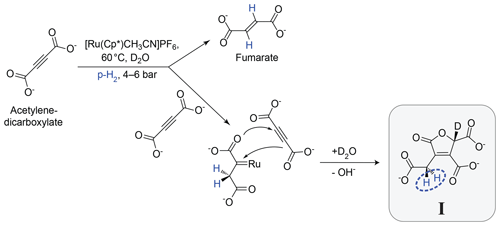Figure 1Postulated mechanism for the formation of I. The main hydrogenation reaction leads to the product fumarate. A side reaction, involving a second acetylenedicarboxylate molecule, leads to the product I. The inequivalent methylene (CH2) protons which derive from para-enriched hydrogen are shown in blue.

I is prone to decomposition and further reactions and could not be isolated and subjected to standard characterization methods. As described in the Supplement, the structure of I was verified by synthesizing a compound with the same structure by an alternative route, followed by a comparison of the 1H NMR spectra.

This paper focuses on the two CH2 protons of the product molecule I which derive from the para-enriched H2 reagent. This proton pair is highlighted in blue in Fig. 1. The chemical equivalence of these CH2 protons is broken by the chiral centre four bonds away, on the opposite side of the five-membered ring.

Figure 2 shows the CH2 region of the 1H NMR spectrum of the reaction product. The black spectrum is the Fourier transform of the NMR signal induced by a single π∕2 pulse, obtained 90 s after the conclusion of the chemical reaction with para-enriched hydrogen. It displays a typical AB pattern, albeit with some spectral intensity distortions from residual hyperpolarization effects (see Appendix B for further explanation). The two protons have a chemical shift difference of Δδ=0.197 ppm and a two-bond J coupling of ${|}^{\mathrm{2}}J|=\mathrm{16.8}$ Hz.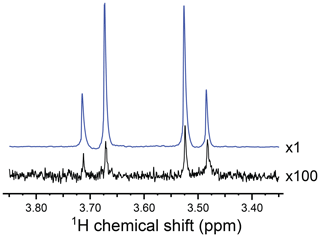Figure 2Partial 1H NMR spectra of the reaction products at a resonance frequency of 400.0 MHz and temperature of 333 K, showing the signals from the CH2 group of I. The hyperpolarized spectrum (blue) was acquired in a gem-PHIP experiment using the pulse sequence in Fig. 3a, with the intervals τ1=90 s and τ2=90 s. The spectrum in black was obtained by waiting 90 s after the conclusion of the experiment and taking the Fourier transform of the NMR signal induced by a single π∕2 pulse. The spectrum shows an AB peak pattern, with intensity distortions from residual hyperpolarization. The AB spectrum is consistent with a chemical shift difference of Δδ=0.197 ppm and a two-bond J coupling of ${|}^{\mathrm{2}}J|=\mathrm{16.8}$ Hz. The signal enhancement factor in the gem-PHIP experiment is estimated to be ∼300, which corresponds to a 1H polarization level of ∼0.9 %.

The nuclear spin relaxation characteristics of I were estimated at room temperature (295 K) and a magnetic field of 9.4 T, using standard techniques (see Supplement). The spin-lattice relaxation time of the CH2 protons is given by ${T}_{\mathrm{1}}=\mathrm{1.23}±\mathrm{0.14}$ s. The singlet relaxation time of the CH2 protons under the same conditions is ${T}_{\text{S}}=\mathrm{61.1}±\mathrm{7.1}$ s. Unfortunately, the chemical instability of I made it difficult to estimate the relaxation times under the much warmer conditions of the gem-PHIP reaction (333 K; see Sect. 5). As described in the Supplement, molecule I decomposes by losing one carboxylic acid group to give an achiral reaction product. The decomposition occurs on a timescale of roughly 2 h at 333 K.

3 Results

## 3.1gem-PHIP

Parahydrogen-induced hyperpolarization of I was demonstrated using the pulse sequence in Fig. 3a. Bubbling of para-enriched hydrogen was conducted for an interval τ1=90 s in the presence of a radiofrequency spin-locking field (), whose frequency corresponds to the mean chemical shift of the CH2 protons. The spin-locking field amplitude corresponded to a 1H nutation frequency of ${\mathit{\omega }}_{\mathrm{nut}}/\mathrm{2}\mathit{\pi }=\mathrm{1.0}$ kHz. Bubbling was switched off and the spin locking continued for a further interval of τ2=30 s. This gave time for the bubbles to dissipate and for a hyperpolarized singlet order to accumulate during the ongoing hydrogenation reaction.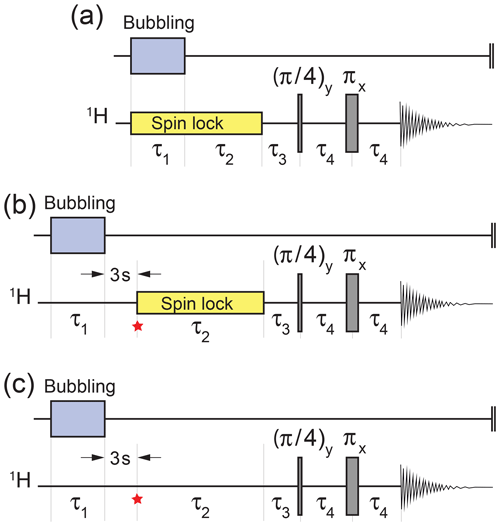Figure 3Experimental timing sequences. (a) Procedure for the demonstration of gem-PHIP. Bubbling of para-enriched H2 is conducted for an interval τ1 in the presence of a radio-frequency spin-locking field in order to suppress singlet–triplet mixing in the reaction product I. The spin locking continues for a further interval τ2, followed by a two-pulse sequence to convert the hyperpolarized singlet order to in-phase magnetization (). The experimental delays were τ3=6.49 ms and τ4=14.97 ms. (b) Procedure for demonstrating the accumulation of the singlet order during spin locking. The spin-lock field is applied during the variable interval τ2, with an amplitude corresponding to a nutation frequency ${\mathit{\omega }}_{\mathrm{nut}}/\left(\mathrm{2}\mathit{\pi }\right)=\mathrm{1.0}$ kHz. The star symbol refers to the time point discussed in the text. (c) The same sequence as for panel (b) but without spin locking during the variable τ2 interval. The interval τ1 was set to 90 s for panel (a) and 17 s for panels (b) and (c).

Hyperpolarized singlet order was converted into in-phase magnetization by the sequence of three delays and two radiofrequency pulses shown in Fig. 3. This sequence converts magnetization into singlet order in weakly coupled spin-1∕2 pairs (). The ideal values of the pulse sequence delays, in the case of infinitely short pulses, are ${\mathit{\tau }}_{\mathrm{3}}=|\mathit{\pi }/{\mathit{\omega }}_{\mathrm{\Delta }}|$ and ${\mathit{\tau }}_{\mathrm{4}}=\mathrm{1}/\left(\mathrm{4}J\right)$, where the chemical shift frequency difference is ωΔ=ω0Δδ and ω0 is the Larmor frequency. In practice, the following pulse sequence intervals were used: τ3=6.49 ms and τ4=14.97 ms.

Figure 2 shows the 1H NMR spectrum of I, hyperpolarized by gem-PHIP (blue spectrum). The NMR signals of the CH2 protons are enhanced by a factor of ∼300 as compared to the spectrum taken 90 s after the end of the pulse sequence (black spectrum). This enhancement factor corresponds to a modest polarization level of ∼0.9 %. Although the achieved polarization level is not spectacular, this experiment demonstrates the feasibility of the gem-PHIP of methylene protons, provided that a spin-locking field is used to stabilize the hyperpolarized singlet order.

## 3.2 Hyperpolarization decay

Figure 4 shows the dependence of the integrated gem-PHIP signal intensity on the spin-locking interval τ2 in Fig. 3a, with the bubbling time τ1 increased to 90 s. Each point in Fig. 4 is the result of an independent experiment, performed on a fresh aliquot of the stock solution, with the signal amplitude normalized against the integrated amplitude of the thermal equilibrium spectrum, obtained 90 s after the pulse sequence has finished. The integrated signal amplitude follows a monoexponential decay function with a time constant of 151±9 s. As discussed below, this time constant may be assigned to the decay time constant TS for a singlet order in the presence of the spin-locking field.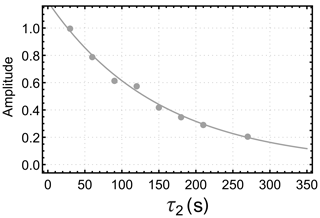Figure 4Dependence of the integrated gem-PHIP signal amplitude for the CH2 protons of I on the interval τ2 in the pulse sequence of Fig. 3a, with τ1 fixed to 90 s. Solid line: fit to Eq. (19) with faAa=1.79, faBa=0 and time constant ${T}_{\mathrm{S}}^{\mathbf{\text{I}}}=\mathrm{151}$ s for singlet-order decay.

## 3.3 Accumulation of a hyperpolarized singlet order

The pulse sequence in Fig. 3b was used to study the accumulation of a hyperpolarized singlet order on the CH2 protons of I during the slow geminal hydrogenation reaction. The experiment started by bubbling para-enriched H2 gas through the NMR tube for τ1=17 s, in order to saturate the solution. The sample was then allowed to rest for a settling time of 3 s in order to dissipate bubbles and to achieve good sample and field homogeneity. The trajectory of the hyperpolarized spin order during the subsequent interval was followed by varying the interval τ2 in a series of independent experiments, each one performed on a separate aliquot of the same stock solution. Experiments were also performed without spin locking during the τ2 interval (Fig. 3c).

The results of this investigation are shown in Fig. 5. When a spin-locking field is applied during the τ2 interval (Fig. 3b), the hyperpolarized signals first increase and then decay (orange symbols). If no spin-locking field is applied during the τ2 interval (Fig. 3c), the hyperpolarized NMR signals decay monotonically with respect to τ2 (blue symbols).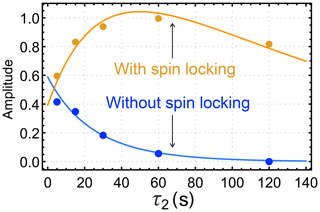Figure 5Dependence of the integrated gem-PHIP signal amplitudes of the CH2 protons of I on the interval τ2 in the pulse sequences of Fig. 3b and c, with τ1 fixed to 17 s. The orange symbols show the amplitudes for the case of a spin-locking field during the τ2 interval (sequence in Fig. 3b), with an amplitude corresponding to the nutation frequency ${\mathit{\omega }}_{\mathrm{nut}}/\mathrm{2}\mathit{\pi }=\mathrm{1.0}$ kHz. The blue symbols show the amplitudes for experiments without a spin-locking field during the τ2 interval (sequence in Fig. 3c). The orange and blue solid lines show the functions ab(τ2) and ac(τ2) (Eqs. 19 and 23, respectively), with the parameters ${T}_{\mathrm{S}}^{\mathbf{\text{I}}}=\mathrm{151}$ s, ${T}_{\mathrm{\Sigma }}^{{\mathrm{H}}_{\mathrm{2}}}=\mathrm{28.7}$ s, ${T}_{zz}^{\mathbf{\text{I}}}=\mathrm{13.2}$ s, ${f}_{b}{C}_{\text{SO}}^{{\mathrm{H}}_{\mathrm{2}}}\left(\mathrm{0}\right)×k=\mathrm{0.059}\phantom{\rule{0.125em}{0ex}}{\mathrm{s}}^{-\mathrm{1}}$, ${f}_{c}{C}_{zz}^{\mathbf{\text{I}}}\left(\mathrm{0}\right)=-\mathrm{1.2}$, and fb=fc.

4 Kinetic analysis

Figure 6 shows the simplified kinetic model which is used to interpret these results. The dynamics of the system may be analysed in terms of the chemical kinetics of the hydrogenation reaction as well as the spin dynamics of the product molecule I. Although the chemical kinetics depend only on concentrations and physical conditions, the spin-dynamical pathways may be manipulated experimentally with fine time resolution, for example by turning spin-locking fields on and off. The experimental results derive from an interplay between the chemical and spin-dynamical domains. Similar analyses have been performed in different contexts ().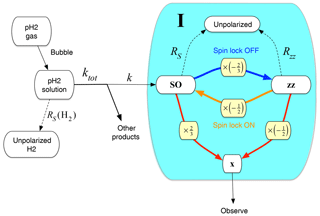Figure 6Kinetic model for gem-PHIP. The chemical reaction of para-enriched H2 with the acetylenedicarboxylate precursor and ruthenium-based catalyst (not shown) proceeds with rate constant k. The shaded area includes the product molecule I in different spin polarization states: unpolarized (top), in a state of singlet nuclear spin order (left), in a state of zz order (right), and with observable x magnetization (bottom). Singlet order decays with rate constant ${R}_{\mathrm{S}}={T}_{\text{S}}^{-\mathrm{1}}$; zz order decays with rate constant ${R}_{zz}={T}_{zz}^{-\mathrm{1}}$. If no spin-locking field is present, singlet order is rapidly converted into zz order, with a conversion factor of $-\mathrm{2}/\mathrm{3}$ (blue arrow and yellow box). If a spin-locking field is applied, zz order is instantaneously projected onto singlet order, with a conversion factor of $-\mathrm{1}/\mathrm{2}$ (orange arrow and yellow box). Singlet order and zz order may be converted into observable x magnetization by the two-pulse sequence in Fig. 3 (red arrows and yellow boxes). The conversion factors in this case are 2∕3 for singlet order and $-\mathrm{1}/\mathrm{2}$ for zz order.

## 4.1 Chemical kinetics

After para-enriched H2 gas is introduced into the solution by bubbling, it starts to react with the acetylenedicarboxylate precursor, catalysed by the ruthenium complex. As depicted in Fig. 1, this is a complex process with the generation of several products and with the production of I requiring an additional precursor molecule. Nevertheless, for the sake of simplicity, and since the acetylenedicarboxylate precursor is in excess, the reaction leading to I is assumed to be first order with respect to the para-H2 reagent and to proceed with rate constant k.

After the bubbling has stopped, the concentrations of the H2 reagent and the product molecule I are assumed to follow the simple kinetic equations:

$\begin{array}{}\text{(1)}& \begin{array}{rl}& \frac{\mathrm{d}}{\mathrm{d}t}{\left[{\mathrm{H}}_{\mathrm{2}}\right]}_{t}=-{k}_{\mathrm{tot}}{\left[{\mathrm{H}}_{\mathrm{2}}\right]}_{t},\\ & \frac{\mathrm{d}}{\mathrm{d}t}{\left[\mathbf{\text{I}}\right]}_{t}=+k{\left[{\mathrm{H}}_{\mathrm{2}}\right]}_{t},\end{array}\end{array}$

where ktot is the rate constant for all hydrogenation reactions, including those that do not lead to the product molecule I, ktot>k. The differential Eq. (1) is easily solved to show that the solution concentration of H2 decays exponentially with time, while the concentration of the product molecule I increases:

$\begin{array}{}\text{(2)}& {\left[{\mathrm{H}}_{\mathrm{2}}\right]}_{t}={\left[{\mathrm{H}}_{\mathrm{2}}\right]}_{\mathrm{0}}\mathrm{exp}\left(-{k}_{\mathrm{tot}}t\right),\text{(3)}& {\left[\mathbf{\text{I}}\right]}_{t}={\left[\mathbf{\text{I}}\right]}_{\mathrm{\infty }}\left(\mathrm{1}-\mathrm{exp}\left(-kt\right)\right),\end{array}$

where the limiting value of the concentration of I is given by

$\begin{array}{}\text{(4)}& {\left[\mathbf{\text{I}}\right]}_{\mathrm{\infty }}=\frac{k}{{k}_{\mathrm{tot}}}{\left[{\mathrm{H}}_{\mathrm{2}}\right]}_{\mathrm{0}}.\end{array}$

As expected, the limiting yield of I depends on the ratio of the productive rate constant k to the total rate constant of all hydrogenation reactions ktot.

## 4.2 Spin dynamics

In this section we discuss some general spin-dynamical scenarios relevant to parahydrogen-enhanced NMR experiments, and in Sect. 4.3 we evaluate the spin dynamics and chemical kinetics specific to the experiments performed in this work. The spin dynamics in this section were evaluated with the assistance of SpinDynamica software ().

### 4.2.1 Singlet order

The proton spins of para-enriched H2 are in a state of enhanced nuclear singlet order, described as the difference between the population of the nuclear singlet state and the mean population of the nuclear triplet states:

$\begin{array}{}\text{(5)}& \mathbf{SO}=〈{S}_{\mathrm{0}}\mathrm{|}\mathit{\rho }\mathrm{|}{S}_{\mathrm{0}}〉-\frac{\mathrm{1}}{\mathrm{3}}\sum _{M=-\mathrm{1}}^{+\mathrm{1}}〈{T}_{M}\mathrm{|}\mathit{\rho }\mathrm{|}{T}_{M}〉,\end{array}$

where ρ is the spin density operator, and the singlet and triplet states are defined in terms of the Zeeman product states as follows ():

$\begin{array}{}\text{(6)}& \begin{array}{rl}& \mathrm{|}{S}_{\mathrm{0}}〉={\mathrm{2}}^{-\mathrm{1}/\mathrm{2}}\left(\mathrm{|}\mathit{\alpha }\mathit{\beta }〉-\mathrm{|}\mathit{\beta }\mathit{\alpha }〉\right),\\ & \mathrm{|}{T}_{+\mathrm{1}}〉=\mathrm{|}\mathit{\alpha }\mathit{\alpha }〉,\\ & \mathrm{|}{T}_{\mathrm{0}}〉={\mathrm{2}}^{-\mathrm{1}/\mathrm{2}}\left(\mathrm{|}\mathit{\alpha }\mathit{\beta }〉+\mathrm{|}\mathit{\beta }\mathit{\alpha }〉\right),\\ & \mathrm{|}{T}_{-\mathrm{1}}〉=\mathrm{|}\mathit{\beta }\mathit{\beta }〉.\end{array}\end{array}$

Singlet order SO may be regarded as the expectation value of the singlet order operator QSO, which is defined as follows:

$\begin{array}{}\text{(7)}& {Q}_{\mathrm{SO}}=\mathrm{|}{S}_{\mathrm{0}}〉〈{S}_{\mathrm{0}}\mathrm{|}-\frac{\mathrm{1}}{\mathrm{3}}\sum _{M=-\mathrm{1}}^{+\mathrm{1}}\mathrm{|}{T}_{M}〉〈{T}_{M}\mathrm{|}=-\frac{\mathrm{4}}{\mathrm{3}}{\mathbf{I}}_{\mathrm{1}}\cdot {\mathbf{I}}_{\mathrm{2}},\end{array}$

so that

$\begin{array}{}\text{(8)}& \mathbf{SO}=〈{Q}_{\mathrm{SO}}〉=\mathrm{Tr}\phantom{\rule{-0.125em}{0ex}}\left\{{Q}_{\mathrm{SO}}^{\mathit{†}}\mathit{\rho }\right\}.\end{array}$

H2 gas in thermal equilibrium at room temperature, with an ortho : para ratio very close to 3 : 1, has a negligible singlet order, SO≃0. Pure para-H2 gas has singlet order SO=1. The current work employs H2 gas which is enriched with the para-spin isomer by thermal equilibration at 77 K. This yields an ortho : para ratio of approximately 1 : 1, corresponding to a singlet order of $\mathbf{SO}\simeq \mathrm{1}/\mathrm{3}$. Assuming that the nuclear spin states are substantially unchanged through the chemical reaction, the product molecule I is formed with its methylene protons in a similar state of finite singlet order, $\mathbf{SO}\simeq \mathrm{1}/\mathrm{3}$.

The singlet-order operator QSO is an exact eigenoperator of the spin propagation superoperator in the case of a magnetically equivalent spin-pair system such as for H2 gas. However, in the product molecule I, the chiral centre breaks the equivalence of the methylene protons, so that the operator QSO is no longer an eigenoperator of the evolution. The chemical shift difference induces singlet–triplet transitions which mix the operator QSO with other operators. However, if a sufficiently strong spin-locking field is applied, the singlet–triplet transitions are suppressed, so that the order SO is substantially unchanged during the evolution, except for a decay due to relaxation processes (). The decay rate constant is given by ${R}_{\text{S}}={T}_{\text{S}}^{-\mathrm{1}}$, where TS is the time constant for singlet-order decay, which is often much longer than the relaxation time constant T1 for longitudinal magnetization. The decay of singlet order in the presence of a spin-locking field, with rate constant RS, is shown in Fig. 6 by the dashed arrow running upwards, connecting the SO state of the reaction product I to the unpolarized state.

### 4.2.2 zz order

A different type of nuclear spin order is called zz order () and corresponds to the expectation value of an operator Qzz defined as follows:

$\begin{array}{}\text{(9)}& \begin{array}{rl}& {Q}_{zz}=\mathrm{2}{I}_{\mathrm{1}z}{I}_{\mathrm{2}z},\\ & \mathbf{zz}=〈{Q}_{zz}〉=\mathrm{Tr}\phantom{\rule{-0.125em}{0ex}}\left\{{Q}_{zz}^{\mathit{†}}\mathit{\rho }\right\}.\end{array}\end{array}$

In the absence of a spin-locking field, and if there is a relatively large chemical shift difference between the coupled spins, the operator Qzz is a better approximation to an eigenoperator of the spin evolution propagator than the singlet-order operator QSO. The relaxation of the system can be complex and multi-exponential in this case. Nevertheless, for the sake of simplicity, we assume here a single rate constant ${R}_{zz}={T}_{zz}^{-\mathrm{1}}$ for the zz order in the absence of a spin-locking field. The time constant Tzz is expected to be close to the ordinary spin-lattice relaxation time constant T1. The decay of zz order in the absence of a spin-locking field, with rate constant Rzz, is shown in Fig. 6 by the dashed arrow running upwards connecting the zz state of the reaction product I to the unpolarized state.

### 4.2.3 Spin locking OFF

Suppose that the molecules of I are in a state of enhanced singlet order SO. This state is stable if a spin-locking field is continuously applied and decays monotonically with the time constant TS. However, if the spin-locking field is turned off, the chemical shift difference between the methylene protons leads to rapid singlet–triplet mixing. The zz-order operator Qzz is an approximate eigenoperator of the evolution in this case, instead of the singlet-order operator QSO. Hence, any singlet order SO which is present when the spin-locking field is turned off is projected onto the zz-order operator Qzz. The remaining spin order corresponds to zero-quantum coherences which rapidly oscillate and decay. These additional components may be ignored to a good approximation, provided that the spin-locking field remains turned off for an interval that is long compared to the difference in chemical shift frequencies.

The zz order created by this projection process is given by

$\begin{array}{}\text{(10)}& \mathbf{zz}=\frac{\mathrm{Tr}\phantom{\rule{-0.125em}{0ex}}\left\{{Q}_{zz}^{\mathit{†}}{Q}_{\mathrm{SO}}\right\}}{\mathrm{Tr}\phantom{\rule{-0.125em}{0ex}}\left\{{Q}_{zz}^{\mathit{†}}{Q}_{zz}\right\}}\mathbf{SO}=-\frac{\mathrm{2}}{\mathrm{3}}\mathbf{SO}.\end{array}$

The projection of SO onto zz is depicted by the blue arrow in Fig. 6, annotated by the projection factor $-\mathrm{2}/\mathrm{3}$ (yellow box).

### 4.2.4 Spin locking ON

Suppose that the molecules of I are in a state of enhanced zz order zz. The corresponding operator Qzz is an eigenoperator of the spin evolution in the absence of a spin-locking field. However, if the spin-locking field is turned on, singlet–triplet mixing is suppressed, and the zz-order operator Qzz is no longer an eigenoperator of the spin evolution. Any zz order which is present when the spin-locking field is turned on is projected onto the singlet-order operator QSO. The remaining spin order corresponds to high-rank spin order terms which rapidly dephase under radiofrequency field inhomogeneity.

The singlet order created by this projection process is given by

$\begin{array}{}\text{(11)}& \mathbf{SO}=\frac{\mathrm{Tr}\phantom{\rule{-0.125em}{0ex}}\left\{{Q}_{\mathrm{SO}}^{\mathit{†}}{Q}_{zz}\right\}}{\mathrm{Tr}\phantom{\rule{-0.125em}{0ex}}\left\{{Q}_{\mathrm{SO}}^{\mathit{†}}{Q}_{\mathrm{SO}}\right\}}\mathbf{zz}=-\frac{\mathrm{1}}{\mathrm{2}}\mathbf{zz}.\end{array}$

The projection of zz onto SO is depicted by the orange arrow in Fig. 6, annotated by the projection factor $-\mathrm{1}/\mathrm{2}$ (yellow box).

The spin orders zz and SO are observed by applying the two-pulse sequence given in Fig. 3c and described in . This sequence converts both types of spin order into observable transverse magnetization, which induces a time-domain NMR signal in the subsequent interval of free precession. The read-out transformations may be written as follows:

$\begin{array}{}\text{(12)}& \begin{array}{rl}& U{Q}_{\mathrm{SO}}{U}^{\mathit{†}}=a\left(\mathbf{SO}\to \mathbf{x}\right){I}_{x}+\mathrm{\dots },\\ & U{Q}_{zz}{U}^{\mathit{†}}=a\left(\mathbf{zz}\to \mathbf{x}\right){I}_{x}+\mathrm{\dots },\end{array}\end{array}$

where U is the propagator for the two-pulse sequence and the dots denote operators which are orthogonal to Ix. These amplitudes may be calculated as follows:

$\begin{array}{}\text{(13)}& \begin{array}{rl}& a\left(\mathbf{SO}\to \mathbf{x}\right)=\frac{\mathrm{Tr}\phantom{\rule{-0.125em}{0ex}}\left\{{I}_{x}^{\mathit{†}}U{Q}_{\mathrm{SO}}{U}^{\mathit{†}}\right\}}{\mathrm{Tr}\phantom{\rule{-0.125em}{0ex}}\left\{{I}_{x}^{\mathrm{2}}\right\}},\\ & a\left(\mathbf{zz}\to \mathbf{x}\right)=\frac{\mathrm{Tr}\phantom{\rule{-0.125em}{0ex}}\left\{{I}_{x}^{\mathit{†}}U{Q}_{zz}{U}^{\mathit{†}}\right\}}{\mathrm{Tr}\phantom{\rule{-0.125em}{0ex}}\left\{{I}_{x}^{\mathrm{2}}\right\}}.\end{array}\end{array}$

In an ideal weakly coupled spin system, with infinitely short, ideal, radiofrequency pulses and delays given by ${\mathit{\tau }}_{\mathrm{3}}=|\mathit{\pi }/{\mathit{\omega }}_{\mathrm{\Delta }}|$ and ${\mathit{\tau }}_{\mathrm{4}}=\mathrm{1}/\left(\mathrm{4}J\right)$, the transformation amplitudes are as follows:

$\begin{array}{}\text{(14)}& \begin{array}{rl}& a\left(\mathbf{SO}\to \mathbf{x}\right)=\frac{\mathrm{2}}{\mathrm{3}},\\ & a\left(\mathbf{zz}\to \mathbf{x}\right)=-\frac{\mathrm{1}}{\mathrm{2}}.\end{array}\end{array}$

These transformations are indicated by the red arrows and yellow boxes in Fig. 6.

The integrated amplitude of the NMR spectrum obtained by Fourier transformation of the NMR signal is therefore proportional to the zz and SO orders before the read-out sequence is applied, multiplied by the transformation factors in Eq. (14).

## 4.3 Analysis of experimental trajectories

The chemical kinetics and spin dynamics may be combined to achieve an understanding of the trajectories in Figs. 4 and 5 generated by the timing sequences shown in Fig. 3.

### 4.3.1 Trajectories with spin locking

The pulse sequences in Fig. 3a, b both examine the dependence of hyperpolarized signals on the duration τ2 of a spin-locking interval. However, the state of the spin system at the start of the τ2 interval is different in the two procedures. In Fig. 3a, which provides the results shown in Fig. 4, spin locking is applied continuously during the bubbling interval and continued during the variable delay τ2. In the sequence of Fig. 3b, on the other hand, which provides the orange data points in Fig. 5, the spin locking is interrupted for 3 s before the τ2 interval starts.

In both cases, the evolution of the singlet order during the spin-locking interval obeys the following differential equations:

$\begin{array}{}\text{(15)}& \begin{array}{rl}& \frac{\mathrm{d}}{\mathrm{d}t}{C}_{\text{SO}}^{{\mathrm{H}}_{\mathrm{2}}}\left(t\right)=-{R}_{\mathrm{\Sigma }}^{{\mathrm{H}}_{\mathrm{2}}}{C}_{\text{SO}}^{{\mathrm{H}}_{\mathrm{2}}}\left(t\right),\\ & \frac{\mathrm{d}}{\mathrm{d}t}{C}_{\text{SO}}^{\mathbf{\text{I}}}\left(t\right)=+k{C}_{\text{SO}}^{{\mathrm{H}}_{\mathrm{2}}}\left(t\right)-{R}_{\mathrm{S}}^{\mathbf{\text{I}}}{C}_{\text{SO}}^{\mathbf{\text{I}}}\left(t\right),\end{array}\end{array}$

The notation ${C}_{\text{SO}}^{X}\left(t\right)$ indicates the total amplitude of singlet spin order for the species X at time point t, taking into account the concentration of X as well as its spin state. The decay rate constant for singlet order in compound I, due to spin-dynamical processes, is denoted ${R}_{\mathrm{S}}^{\mathbf{\text{I}}}={T}_{\text{S}}\left(\mathbf{\text{I}}{\right)}^{-\mathrm{1}}$. The total decay rate constant for H2 singlet order, due to the combination of chemical and spin-dynamical processes, is denoted

$\begin{array}{}\text{(16)}& {R}_{\mathrm{\Sigma }}^{{\mathrm{H}}_{\mathrm{2}}}={k}_{\mathrm{tot}}+{R}_{\mathrm{S}}^{{\mathrm{H}}_{\mathrm{2}}},\end{array}$

where ${R}_{\mathrm{S}}^{{\mathrm{H}}_{\mathrm{2}}}$ denotes the decay rate constant for H2 singlet order, due to para-to-ortho conversion in solution, in the presence of the hydrogenation catalyst but in the absence of a hydrogenation reaction. Note that this rate constant may be greatly increased by the presence of the catalyst, since transient binding of H2 molecules with the catalyst provides an efficient mechanism for orthopara conversion.

Equation (15) may be solved to obtain the following trajectory of the singlet order for compound I under spin locking:

$\begin{array}{}\text{(17)}& {C}_{\text{SO}}^{\mathbf{\text{I}}}\left({\mathit{\tau }}_{\mathrm{2}},i\right)={A}_{i}\mathrm{exp}\left\{-{R}_{\mathrm{S}}^{\mathbf{\text{I}}}{\mathit{\tau }}_{\mathrm{2}}\right\}+{B}_{i}\mathrm{exp}\left\{-{R}_{\mathrm{\Sigma }}^{{\mathrm{H}}_{\mathrm{2}}}{\mathit{\tau }}_{\mathrm{2}}\right\},\end{array}$

where the coefficients are

$\begin{array}{}\text{(18)}& \begin{array}{rl}& {A}_{i}={C}_{\text{SO}}^{\mathbf{\text{I}}}\left(\mathrm{0},i\right)-{B}_{i},\\ & {B}_{i}=\frac{k{C}_{\text{SO}}^{{\mathrm{H}}_{\mathrm{2}}}\left(\mathrm{0},i\right)}{{R}_{\mathrm{S}}^{\mathbf{\text{I}}}-{R}_{\mathrm{\Sigma }}^{{\mathrm{H}}_{\mathrm{2}}}}.\end{array}\end{array}$

The index i refers to the first two pulse sequences in Fig. 3, $i\in \mathit{\left\{}a,b\mathit{\right\}}$. The symbol ${C}_{\text{SO}}^{\mathbf{\text{I}}}\left(\mathrm{0},i\right)$ is the total amplitude of H2 singlet order at the start of the spin-lock interval in experiment i, taking into account the concentration of I as well as its spin state.

The amplitude factor for the read-out of a singlet order is given by $\left(+\mathrm{2}/\mathrm{3}\right)$, as shown by Eq. (14). Hence the integrated signal amplitudes for the sequences in Fig. 3a, b are given by

$\begin{array}{}\text{(19)}& {a}_{i}\left({\mathit{\tau }}_{\mathrm{2}}\right)=\frac{\mathrm{2}}{\mathrm{3}}{f}_{i}\left({A}_{i}\mathrm{exp}\left\{-{R}_{\mathrm{S}}^{\mathbf{\text{I}}}{\mathit{\tau }}_{\mathrm{2}}\right\}+{B}_{i}\mathrm{exp}\left\{-{R}_{\mathrm{\Sigma }}^{{\mathrm{H}}_{\mathrm{2}}}{\mathit{\tau }}_{\mathrm{2}}\right\}\right),\end{array}$

where fi are instrumental factors and $i\in \mathit{\left\{}a,b\mathit{\right\}}$. The signal trajectories have a bi-exponential form, in general.

### 4.3.2 Trajectory without spin locking

The sequence in Fig. 3c is identical to that in Fig. 3b, except for the absence of the spin-locking field during the τ2 interval. In the absence of spin locking, the relevant eigenoperator of the spin evolution during the τ2 interval is the zz operator Qzz (Eq. 9). The combined chemical–spin dynamics of the system is described by the following differential equations:

$\begin{array}{}\text{(20)}& \begin{array}{rl}& \frac{\mathrm{d}}{\mathrm{d}t}{C}_{\text{SO}}^{{\mathrm{H}}_{\mathrm{2}}}\left(t\right)=-{R}_{\mathrm{\Sigma }}^{{\mathrm{H}}_{\mathrm{2}}}{C}_{\text{SO}}^{{\mathrm{H}}_{\mathrm{2}}}\left(t\right),\\ & \frac{\mathrm{d}}{\mathrm{d}t}{C}_{zz}^{\mathbf{\text{I}}}\left(t\right)=\left(-\frac{\mathrm{2}}{\mathrm{3}}\right)k{C}_{\text{SO}}^{{\mathrm{H}}_{\mathrm{2}}}\left(t\right)-{R}_{zz}^{\mathbf{\text{I}}}{C}_{zz}^{\mathbf{\text{I}}}\left(t\right),\end{array}\end{array}$

The factor $\left(-\mathrm{2}/\mathrm{3}\right)$ appears since the H2 singlet order is projected onto the zz order of the product molecule I upon hydrogenation, as described in Sect. 4.2.3. This equation is valid provided that the interval t exceeds the time required for dephasing of spin-order components orthogonal to zz order after hydrogenation in the absence of a spin-locking field.

The differential Eq. (20) may be solved to obtain the following trajectory for the zz order of compound I, under the pulse sequence of Fig. 3c:

$\begin{array}{}\text{(21)}& {C}_{zz}^{\mathbf{\text{I}}}\left(t,c\right)={A}_{c}\mathrm{exp}\left\{-{R}_{zz}^{\mathbf{\text{I}}}t\right\}+{B}_{c}\mathrm{exp}\left\{-{R}_{\mathrm{\Sigma }}^{{\mathrm{H}}_{\mathrm{2}}}t\right\},\end{array}$

where the coefficients are

$\begin{array}{}\text{(22)}& \begin{array}{rl}& {A}_{c}={C}_{zz}^{\mathbf{\text{I}}}\left(\mathrm{0},c\right)-{B}_{c},\\ & {B}_{c}=-\frac{\mathrm{2}k{C}_{\text{SO}}^{{\mathrm{H}}_{\mathrm{2}}}\left(\mathrm{0},c\right)}{\mathrm{3}\left({R}_{zz}^{\mathbf{\text{I}}}-{R}_{\mathrm{\Sigma }}^{{\mathrm{H}}_{\mathrm{2}}}\right)}.\end{array}\end{array}$

Here ${C}_{zz}^{\mathbf{\text{I}}}\left(\mathrm{0},c\right)$ is the zz order of compound I at the beginning of the τ2 interval. This equation assumes that t is longer than the time required for dephasing of spin-order components orthogonal to the singlet order, in the presence of the spin-locking field. The zz order at the end of the τ2 interval is transformed into observable x magnetization by applying a sequence of two pulses and three delays. The amplitude factor for the read-out of zz order is given by $\left(-\mathrm{1}/\mathrm{2}\right)$, as shown by Eq. (14). Hence the integrated signal amplitude for the sequence in Fig. 3c is proportional to

$\begin{array}{}\text{(23)}& {a}_{c}\left({\mathit{\tau }}_{\mathrm{2}}\right)=-\frac{\mathrm{1}}{\mathrm{2}}{f}_{c}\left({A}_{c}\mathrm{exp}\left\{-{R}_{zz}^{\mathbf{\text{I}}}{\mathit{\tau }}_{\mathrm{2}}\right\}+{B}_{c}\mathrm{exp}\left\{-{R}_{\mathrm{\Sigma }}^{{\mathrm{H}}_{\mathrm{2}}}{\mathit{\tau }}_{\mathrm{2}}\right\}\right).\end{array}$

This also has the form of a bi-exponential decay.

Since the sequences in Fig. 3b and c are the same up to the start of the τ2 interval (indicated by the star in the pulse-sequence diagrams), the instrumental factors are identical (fb=fc) and we can write

$\begin{array}{}\text{(24)}& {C}_{\text{SO}}^{\mathbf{\text{I}}}\left(\mathrm{0},b\right)=-\frac{\mathrm{1}}{\mathrm{2}}{C}_{zz}^{\mathbf{\text{I}}}\left(\mathrm{0},c\right)\end{array}$

using the projection in Eq. (11). Hence the signal amplitudes for these two experiments have the following relationship when extrapolated back to the start of the τ2 interval:

$\begin{array}{}\text{(25)}& \frac{{\mathit{\alpha }}_{b}\left(\mathrm{0}\right)}{{\mathit{\alpha }}_{c}\left(\mathrm{0}\right)}=\frac{\mathrm{2}}{\mathrm{3}}.\end{array}$

The difference in extrapolated starting points is evident in the theoretical curves shown by the solid lines in Fig. 5.

Since the procedures in Fig. 3b and c are identical for τ2=0, one would expect αb(0)=αc(0), in contradiction to Eq. (25). This apparent paradox is resolved by noting that the derivation of Eq. (25) relies on the projections in Eqs. (10) and (11), which are not valid for very short intervals τ2.

### 4.3.3 Data fitting

The data sets of Figs. 4 and 5 were fitted simultaneously using the set of global parameters. All three data sets were well fitted by the functions aa(τ2), ab(τ2), and ac(τ2) (Eqs. 19 and 23) with the following parameters: ${T}_{\mathrm{S}}^{\mathbf{\text{I}}}=\mathrm{151}±\mathrm{9}$ s; ${T}_{\mathrm{\Sigma }}^{{\mathrm{H}}_{\mathrm{2}}}=\mathrm{28.7}±\mathrm{3.8}$ s; ${T}_{zz}^{\mathbf{\text{I}}}=\mathrm{13.2}±\mathrm{1.3}$ s; ${f}_{a}{A}_{a}=\mathrm{1.79}±\mathrm{0.07}$; fbBa≈0; ${f}_{b}{C}_{\text{SO}}^{{\mathrm{H}}_{\mathrm{2}}}\left(\mathrm{0},b\right)×k=\mathrm{0.059}±\mathrm{0.007}\phantom{\rule{0.125em}{0ex}}{\mathrm{s}}^{-\mathrm{1}}$; ${f}_{c}{C}_{zz}^{\mathbf{\text{I}}}\left(\mathrm{0},c\right)=-\mathrm{1.2}±\mathrm{0.1}$. All rate constants are expressed here as time constants, i.e. ${T}_{X}={R}_{X}^{-\mathrm{1}}$. The parameters ${f}_{b}{C}_{\text{SO}}^{{\mathrm{H}}_{\mathrm{2}}}\left(\mathrm{0},b\right)$ and k interact strongly in the fit and could not be determined independently. The coefficient of determination R2 was estimated to be 0.991 for the fit in Fig. 4 and 0.925 and 0.966 for the fits of the build-up and decay curves in Fig. 5, respectively.

For these parameters, the trajectory in Fig. 4 is very close to a single-exponential decay with time constant ${T}_{\mathrm{S}}^{\mathbf{\text{I}}}=\mathrm{151}±\mathrm{9}$ s. For the case of the orange curve in Fig. 5, on the other hand, the singlet order on I starts at a relatively low level. The long singlet decay time constant allows accumulation of a singlet order as the reaction proceeds in the presence of the spin-locking field. This accumulation gives rise to the rising initial trajectory of the orange curve in Fig. 5. The comparatively short time constant for the decay of zz order, ${T}_{zz}^{\mathbf{\text{I}}}\simeq \mathrm{13.2}$ s, allows no time for zz order to accumulate in the absence of a spin-locking field, giving rise to the monotonically decaying blue curve in Fig. 5.

The singlet decay time constant for the methylene protons of compound I was determined independently by non-hyperpolarized experiments (see Supplement). These experiments were performed at a much lower sample temperature of 295 K to avoid the decomposition of I. The estimated value of ${T}_{\mathrm{S}}^{\mathbf{\text{I}}}$ at 295 K and a magnetic field of 9.41 T is 61.1±7.1 s. This value is much shorter than the estimate of ${T}_{\mathrm{S}}^{\mathbf{\text{I}}}=\mathrm{151}±\mathrm{9}$ s from the hyperpolarization trajectories. The discrepancy may be due in part to a reduction in rotational correlation time for the molecules of I at the elevated temperature used in the PHIP experiments.

5 Materials and methods

All experiments were conducted on a Bruker Avance Neo 400 MHz (9.41 T) system equipped with a 5 mm BBO probe. The excitation pulses were applied on-resonance with the doublet at 3.6 to 3.7 ppm. Their amplitude corresponded to a nutation frequency of ∼20 kHz. The spectral width was set to 20 ppm with sampling of 65k points.

The reagent solution consisted of 100 mM disodium acetylenedicarboxylate and 6 mM [CpRu(CH3CN)3]PF6 dissolved in D2O. All sample solutions were prepared by mixing the components, sonicating the mixture for 5 min at 50 C and filtering it through a 0.2 µm pore-size syringe filter with a nylon membrane.

Para-enriched hydrogen was produced by slowly passing hydrogen gas through an iron oxide catalyst submerged in liquid nitrogen to obtain 50 % para-enriched hydrogen. A container was pressurized with 10 bar of para-enriched H2 to contain gas for a whole series of experiments at 4 bar of parahydrogen pressure.

Hydrogenation experiments of disodium acetylenedicarboxylate and catalyst [CpRu(CH3CN)3]PF6 were carried out strictly according to the experimental procedure in Table 1. For each experiment, a 300 µL aliquot was used from a stock solution. Bubbling was performed in a 5 mm Wilmad® quick pressure valve NMR tube through a $\mathrm{1}/{\mathrm{16}}^{\prime \prime }$ PEEK capillary, using 4 bar parahydrogen pressure, 60 C (333 K) temperature, and a gas flow of 400 sccm.

Spin locking was performed by irradiating a continuous wave rf field at the mean resonance frequency of the CH2 protons and with an amplitude corresponding to a 1.0 kHz nutation frequency.

6 Conclusions

In this work we have demonstrated geminal hydrogenation of a precursor molecule using para-enriched hydrogen gas. We show that singlet order for the methylene proton pair may be maintained by application of a spin-locking field and that the proton singlet order in the product molecule relaxes with the time constant ${T}_{\mathrm{S}}^{\mathbf{\text{I}}}\simeq \mathrm{151}$ s, which is more than 50 times T1. We have developed a simplified kinetic model to describe the time dependence of the hyperpolarized signals observed in such experiments, which include the chemical kinetics as well as the spin dynamics. This allows simultaneous fitting of the data from several experiments and estimation of most of the kinetic parameters and relaxation rate constants.

The particular hydrogenation reaction discussed here does not lead to a product molecule with a biological function. Nevertheless, our results demonstrate the principle of methylene hyperpolarization by hydrogenative PHIP and that the short T1 values of these protons do not necessarily prevent the accumulation of hyperpolarization. We hope that this work might allow exploration of a new range of hyperpolarized molecular targets.

Appendix A:1H NMR spectrum of the reaction solution

The 1H NMR spectrum of the reaction solution, together with peak assignments, is given in Fig. A1.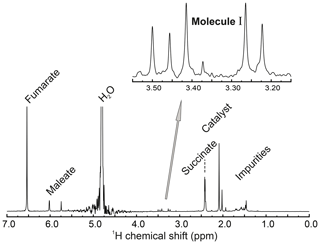Figure A1Full 1H NMR spectrum (400 MHz) of the reaction solution after hydrogenation, taken at a temperature of 298 K. An expanded view of the methylene region is shown. This shows four peaks from the inequivalent methylene protons of the reaction product I as well as an additional peak at 3.50 ppm from the achiral decomposition product of I (see Supplement). The small peak at 3.37 ppm is unassigned. The spectrum shows peaks from several other substances, including fumarate, succinate, and maleate, as well as the hydrogenation catalyst.

Appendix B: Partially relaxed hyperpolarized spectra

Figure 2 shows two 1H NMR spectra. The first was taken immediately after a PHIP experiment, while the second was taken after waiting for an interval of 90 s after the PHIP experiment. A minor asymmetry in the “roof effect” pattern of line amplitudes may be seen in the latter spectrum. An explanation of this observation is provided below.

The coherent spin Hamiltonian for a system of two chemically inequivalent protons may be written as follows:

$\begin{array}{}\text{(B1)}& \begin{array}{rl}& {\mathcal{H}}_{\mathrm{coh}}={\mathit{\omega }}_{\mathrm{\Delta }}\left({I}_{\mathrm{1}z}-{I}_{\mathrm{2}z}\right)+\mathrm{2}\mathit{\pi }J{\mathbf{I}}_{\mathrm{1}}\cdot {\mathbf{I}}_{\mathrm{2}},\\ & {\mathit{\omega }}_{\mathrm{\Delta }}=-{\mathit{\gamma }}_{H}{B}_{\mathrm{0}}\left({\mathit{\delta }}_{\mathrm{1}}-{\mathit{\delta }}_{\mathrm{2}}\right).\end{array}\end{array}$

This Hamiltonian is expressed in a rotating frame at the mean resonance frequency of the two spins. The parameters for numerical simulations were set to J=16.9 Hz and ${\mathit{\omega }}_{\mathrm{\Delta }}/\mathrm{2}\mathit{\pi }=\mathrm{80}$ Hz. Numerical simulations were performed using SpinDynamica software ().

Two different initial density matrices were considered: (1) ρ1=Iz, corresponding to longitudinal magnetization, neglecting the unity operator and numerical factors for simplicity. (2) The definition of the second density operator is more complex. The reaction with para-enriched hydrogen deposits proton singlet order in the reaction product which evolves under the Hamiltonian of the new system. Since the singlet order is not an eigenoperator of the spin evolution, a secular approximation must be applied. This omits any coherences created by instant projection of the singlet order onto the Hamiltonian eigenbasis. Thus, the second density matrix ρ2 corresponds to the singlet order of the two protons secularized according to the Hamiltonian in Eq. (B1), with the coupling and chemical shift values given above.

A strong π∕2 pulse excites very different spectra for the two initial density operators. The first spectrum displays a distinct “roof effect” for the peak amplitudes, whereas the second spectrum displays an alternating peak pattern, typical for parahydrogen-enhanced NMR (). If the sample contains a fully relaxed spin ensemble together with a similar amount of singlet order originating from a previous parahydrogen reaction, the total spectrum is a superposition of the two different patterns (see Fig. B1). This gives a good match to the experimental spectrum in Fig. 2.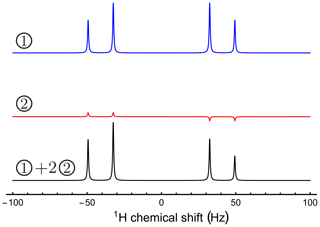Figure B1The origin of the asymmetry observed in Fig. 2. The simulated spectra show signals generated by two different density operators, excited by an identical π∕2 pulse. The first initial state corresponds to pure longitudinal magnetization. The second initial state corresponds to a singlet order secularized under the Hamiltonian given in Eq. (B1). A suitably weighted superposition of the two spectra is a good match to the experimental spectrum.

Data availability
Data availability.

All 1H NMR spectra, the general pulse sequence used, as well as data points seen in the kinetics are openly available from the University of Southampton repository at https://doi.org/10.5258/SOTON/D1494 .

Supplement
Supplement.

Author contributions
Author contributions.

LD prepared, carried out, and optimized the experiments, did the data analysis and fitting of data, supported the kinetic model development, and wrote the manuscript. BR prepared and carried out the experiments, coordinated the project, and wrote the manuscript. ML postulated the chemical structure of I and the reaction path, proposed independent synthesis of I for its chemical structure determination, consulted in the interpretation of NMR data, and contributed to the writing of the manuscript. GAIM carried out the independent synthesis of I and determined its chemical structure, consulted in the interpretation of NMR spectra and in the identification of chemical degradation processes, and contributed to the writing of the Supplement. JE performed the first experiments, which indicated a hyperpolarized singlet state on the methylene protons of I, consulted in experimental design and data fitting, supported the kinetic model development, and wrote the manuscript. JFPC did the experimental setup engineering for finely adjustable and reproducible experiments, consulted in experimental design, interpreted NMR spectra, identified the chemical degradation processes, and wrote the manuscript. MHL did the scientific supervision and idea generation, consulted in NMR pulse-sequence design and the theoretical background, developed the kinetic models, fitted the data, and wrote the manuscript.

Competing interests
Competing interests.

The authors declare that they have no conflict of interest.

Acknowledgements
Acknowledgements.

This project was funded by the Marie Skłodowska-Curie programme of the European Union (grant no. 766402), the European Research Council (786707 – FunMagResBeacons), and EPSRC-UK (grant nos. EP/P009980/1 and EP/P030491/1). Markus Leutzsch acknowledges generous support from the Max Planck Society.

Financial support
Financial support.

This research has been supported by the H2020 Marie Skłodowska-Curie Actions (ZULF; grant no. 766402), the EPSRC-UK (grant nos. EP/P009980/1 and EP/P030491/1), and the European Research Council (FunMagResBeacons grant no. 786707).

Review statement
Review statement.

This paper was edited by Konstantin Ivanov and reviewed by two anonymous referees.

References

Adams, R. W., Aguilar, J. A., Atkinson, K. D., Cowley, M. J., Elliott, P. I. P., Duckett, S. B., Green, G. G. R., Khazal, I. G., Lopez-Serrano, J., and Williamson, D. C.: Reversible Interactions with Para-Hydrogen Enhance NMR Sensitivity by Polarization Transfer, Science, 323, 1708–1711, 2009. a, b

Ardenkjaer-Larsen, J. H., Fridlund, B., Gram, A., Hansson, G., Hansson, L., Lerche, M. H., Servin, R., Thaning, M., and Golman, K.: Increase in Signal-to-Noise Ratio of >10 000 Times in Liquid-State NMR, P. Natl. Acad. Sci. USA, 100, 10158–10163, 2003. a

Barskiy, D. A., Knecht, S., Yurkovskaya, A. V., and Ivanov, K. L.: SABRE: Chemical Kinetics and Spin Dynamics of the Formation of Hyperpolarization, Prog. Nucl. Mag. Res. Sp., 114–115, 33–70, 2019. a

Bengs, C. and Levitt, M. H.: SpinDynamica: Symbolic and Numerical Magnetic Resonance in a Mathematica Environment, Magn. Reson. Chem., 56, 374–414, 2018. a, b

Bowers, C. R. and Weitekamp, D. P.: Parahydrogen and Synthesis Allow Dramatically Enhanced Nuclear Alignment, J. Am. Chem. Soc., 109, 5541–5542, 1987. a, b, c, d

Carravetta, M. and Levitt, M. H.: Long-Lived Nuclear Spin States in High-Field Solution NMR, J. Am. Chem. Soc., 126, 6228–6229, 2004. a, b, c

Carravetta, M. and Levitt, M. H.: Theory of Long-Lived Nuclear Spin States in Solution Nuclear Magnetic Resonance. I. Singlet States in Low Magnetic Field, J. Chem. Phys., 122, 214505, https://doi.org/10.1063/1.1893983, 2005. a

Carravetta, M., Johannessen, O. G., and Levitt, M. H.: Beyond the T1 Limit: Singlet Nuclear Spin States in Low Magnetic Fields, Phys. Rev. Lett., 92, 153003, https://doi.org/10.1103/PhysRevLett.92.153003, 2004. a, b

Dagys, L., Ripka, B., Leutzsch, M., Moustafa, G. A. I., Eills, J., Colell, J. F. P., and Levitt, M. H.: Data set for Geminal Parahydrogen-Induced Polarization: Accumulating Long-Lived Singlet Order on Methylene Proton Pairs, University of Southampton repository, https://doi.org/10.5258/SOTON/D1494, 2020. a

Eills, J., Cavallari, E., Carrera, C., Budker, D., Aime, S., and Reineri, F.: Real-Time Nuclear Magnetic Resonance Detection of Fumarase Activity Using Parahydrogen-Hyperpolarized [1-13C]Fumarate, J. Am. Chem. Soc., 141, 20209–20214, 2019. a

Emondts, M., Colell, J. F. P., Blümich, B., and Schleker, P. P. M.: Polarization Transfer Efficiency in PHIP Experiments, Phys. Chem. Chem. Phys., 19, 21933–21937, 2017. a

Fürstner, A.: Trans-Hydrogenation, Gem-Hydrogenation, and Trans-Hydrometalation of Alkynes: An Interim Report on an Unorthodox Reactivity Paradigm, J. Am. Chem. Soc., 141, 11–24, 2019. a

Goez, M.: Chapter 3 Photo-CIDNP Spectroscopy, in: Annual Reports on NMR Spectroscopy, vol. 66, 77–147, Academic Press, London, UK, 2009. a

Gopalakrishnan, K. and Bodenhausen, G.: Lifetimes of the Singlet-States under Coherent off-Resonance Irradiation in NMR Spectroscopy, J. Magn. Reson., 182, 254–259, 2006. a

Guthertz, A., Leutzsch, M., Wolf, L. M., Gupta, P., Rummelt, S. M., Goddard, R., Farès, C., Thiel, W., and Fürstner, A.: Half-Sandwich Ruthenium Carbene Complexes Link Trans-Hydrogenation and Gem-Hydrogenation of Internal Alkynes, J. Am. Chem. Soc., 140, 3156–3169, 2018. a, b, c

Harthun, A., Selke, R., and Bargon, J.: Proof of a Reversible, Pairwise Hydrogen Transfer during the Homogeneously Rhodium(I)-Catalyzed Hydrogenation of α,β-Unsaturated Carbonic Acid Derivatives with In Situ NMR Spectroscopy and Parahydrogen, Angew. Chem. Int. Edit., 35, 2505–2507, 1996. a

Hübler, P., Giernoth, R., Kümmerle, G., and Bargon, J.: Investigating the Kinetics of Homogeneous Hydrogenation Reactions Using PHIP NMR Spectroscopy, J. Am. Chem. Soc., 121, 5311–5318, 1999. a

Hübler, P., Bargon, J., and Glaser, S. J.: Nuclear Magnetic Resonance Quantum Computing Exploiting the Pure Spin State of Para Hydrogen, J. Chem. Phys., 113, 2056–2059, 2000. a, b

Kaptein, R.: Chemically Induced Dynamic Nuclear Polarization. VIII. Spin Dynamics and Diffusion of Radical Pairs, J. Am. Chem. Soc., 94, 6251–6262, 1972. a

Kiryutin, A. S., Panov, M. S., Yurkovskaya, A. V., Ivanov, K. L., and Bodenhausen, G.: Proton Relaxometry of Long-Lived Spin Order, ChemPhysChem, 20, 766–772, 2019. a

Kovtunov, K. V., Pokochueva, E. V., Salnikov, O. G., Cousin, S. F., Kurzbach, D., Vuichoud, B., Jannin, S., Chekmenev, E. Y., Goodson, B. M., Barskiy, D. A., and Koptyug, I. V.: Hyperpolarized NMR Spectroscopy: D-DNP, PHIP, and SABRE Techniques, Chemistry – An Asian Journal, 13, 1857–1871, 2018. a, b

Leutzsch, M., Wolf, L. M., Gupta, P., Fuchs, M., Thiel, W., Farès, C., and Fürstner, A.: Formation of Ruthenium Carbenes by Gem-Hydrogen Transfer to Internal Alkynes: Implications for Alkyne Trans-Hydrogenation, Angew. Chem. Int. Edit., 54, 12431–12436, 2015. a, b

Levitt, M. H.: Singlet Nuclear Magnetic Resonance, Annu. Rev. Phys. Chem., 63, 89–105, 2012. a, b

Levitt, M. H.: Long Live the Singlet State!, J. Magn. Res., 306, 69–74, 2019. a

Lindale, J. R., Eriksson, S. L., Tanner, C. P. N., Zhou, Z., Colell, J. F. P., Zhang, G., Bae, J., Chekmenev, E. Y., Theis, T., and Warren, W. S.: Unveiling Coherently Driven Hyperpolarization Dynamics in Signal Amplification by Reversible Exchange, Nat. Commun., 10, 1–7, 2019. a, b

Maly, T., Debelouchina, G. T., Bajaj, V. S., Hu, K.-N., Joo, C.-G., Mak-Jurkauskas, M. L., Sirigiri, J. R., van der Wel, P. C. A., Herzfeld, J., Temkin, R. J., and Griffin, R. G.: Dynamic Nuclear Polarization at High Magnetic Fields, J. Chem. Phys., 128, 052211, https://doi.org/10.1063/1.2833582, 2008.  a

Natterer, J. and Bargon, J.: Parahydrogen Induced Polarization, Prog. Nucl. Magn. Res. Sp., 31, 293–315, 1997. a, b

Pileio, G. and Levitt, M. H.: Theory of Long-Lived Nuclear Spin States in Solution Nuclear Magnetic Resonance. II. Singlet Spin Locking, J. Chem. Phys., 130, 214501, https://doi.org/10.1063/1.3139064, 2009. a, b

Pileio, G., Carravetta, M., and Levitt, M. H.: Storage of Nuclear Magnetization as Long-Lived Singlet Order in Low Magnetic Field, P. Natl. Acad. Sci. USA, 107, 17135–17139, 2010. a

Pravdivtsev, A. N., Ivanov, K. L., Yurkovskaya, A. V., Petrov, P. A., Limbach, H.-H., Kaptein, R., and Vieth, H.-M.: Spin Polarization Transfer Mechanisms of SABRE: A Magnetic Field Dependent Study, J. Magn. Res., 261, 73–82, 2015. a

Reineri, F., Boi, T., and Aime, S.: ParaHydrogen Induced Polarization of 13C Carboxylate Resonance in Acetate and Pyruvate, Nat. Commun., 6, 5858, https://doi.org/10.1038/ncomms6858, 2015. a

Ripka, B., Eills, J., Kouřilová, H., Leutzsch, M., Levitt, M. H., and Münnemann, K.: Hyperpolarized Fumarate via Parahydrogen, Chem. Commun., 54, 12246–12249, 2018. a, b, c

Sarkar, R., Vasos, P. R., and Bodenhausen, G.: Singlet-State Exchange NMR Spectroscopy for the Study of Very Slow Dynamic Processes, J. Am. Chem. Soc., 129, 328–334, 2007. a, b, c

Song, L., Feng, Q., Wang, Y., Ding, S., Wu, Y.-D., Zhang, X., Chung, L. W., and Sun, J.: Ru-Catalyzed Migratory Geminal Semihydrogenation of Internal Alkynes to Terminal Olefins, J. Am. Chem. Soc., 141, 17441–17451, 2019. a, b

Sørensen, O. W., Eich, G. W., Levitt, M. H., Bodenhausen, G., and Ernst, R. R.: Product Operator Formalism for the Description of NMR Pulse Experiments, Prog. Nucl. Magn. Res. Sp., 16, 163–192, 1984. a

Theis, T., Truong, M., Coffey, A. M., Chekmenev, E. Y., and Warren, W. S.: LIGHT-SABRE Enables Efficient in-Magnet Catalytic Hyperpolarization, J. Magn. Res., 248, 23–26, 2014. a

Truong, M. L., Theis, T., Coffey, A. M., Shchepin, R. V., Waddell, K. W., Shi, F., Goodson, B. M., Warren, W. S., and Chekmenev, E. Y.: 15N Hyperpolarization by Reversible Exchange Using SABRE-SHEATH, J. Phys. Chem. C, 119, 8786–8797, 2015. a

Walker, T. G. and Happer, W.: Spin-Exchange Optical Pumping of Noble-Gas Nuclei, Rev. Mod. Phys., 69, 629–642, 1997. a

Zhang, G., Colell, J. F. P., Glachet, T., Lindale, J. R., Reboul, V., Theis, T., and Warren, W. S.: Terminal Diazirines Enable Reverse Polarization Transfer from 15N2 Singlets, Angew. Chem., 131, 11235–11241, 2019. a, b